# Numerical and Experimental Study of Natural Convection in a Tunnel Greenhouse Located in South West Algeria (Adrar Region)

Numerical and Experimental Study of Natural Convection in a Tunnel Greenhouse Located in South West Algeria (Adrar Region)

Mohammed Salah BelalemMohammed Elmir Mohammed Tamali Razli Mehdaoui Abdelkrim Missoum Toufik Chergui Salah Bezari

Thermo-fluidics Research Team, Energetics in Arid Zones Laboratory (ENERGARID), Faculty of Technology, TAHRI Mohamed University of Bechar, P.O.Box 417, Bechar, Algeria

Mechanics, Modeling and Experimentation Laboratory (L2ME), Faculty of Technology, TAHRI Mohamed University of Bechar, P.O.Box 417, Bechar, Algeria

Renewable Energy Applied Research Unit (URAER), CDER, Ghardaia 47133, Algeria

Corresponding Author Email:
belalemmedsalah@yahoo.com
Page:
1575-1582
|
DOI:
https://doi.org/10.18280/ijht.390520
7 January 2021
|
Accepted:
18 September 2021
|
Published:
31 October 2021
| Citation

OPEN ACCESS

Abstract:

In this work, we propose to experimentally and numerically study the natural convection in laminar regime in an agricultural greenhouse located in South West of Algeria and more precisely in the Adrar area. The numerical study is two-dimensional and was carried out on a tunnel greenhouse with an area of 180m2 located in Adrar in the southwest of Algeria (Latitude: 27°52′27″N, longitude: 0°17′37″W, the laltitude above sea level is 257 m), with polyethylene cover and houses two rows of tomato plants. The experimental study was made during the winter or flowering period of tomato plants (February) when the temperature difference outside the greenhouse is maximum: T min = 3℃ at night and T max = 20℃ the day. We used a calculate code based on the finite element method to numerically simulate the phenomenon of heat transfer inside the greenhouse. The results of the numerical simulation are in the form of isotherms, streamlines and variations in temperature and speed in the greenhouse. The value of the temperature calculated by numerical simulation at the position where the sensor has been placed will be compared with that measured by the sensor. It was concluded that to have a favorable environment for the growth of tomatoes, we must keep the openings closed especially during the night without needing a heating system, especially in this region characterized by a hyper arid climate.

Keywords:

greenhouse, plant, tomato, sensor, porous medium, natural convection, numerical simulation, finite elements method

1. Introduction

The main objective of our work is to numerically study the distribution of hydrodynamic and thermal fields inside the tunnel greenhouse placed in the arid region of Adrar which is characterized by a dry climate (5℃ in winter and +50℃ in summer). We use a numerical code based on the finite element method to resolve the differential partial equations (DPE) of hydrodynamics and heat transfer inside the greenhouse.

This makes this work a contribution to the determination and visualization of heat transfer mechanisms inside agricultural greenhouses used massively in the last decade to develop and promote greenhouse cultivation and in particular production local tomato; seeing that the latter is a crop that has been done in the open fields for centuries by different generations of farmers in Adrar.

2. Materials and Methods

2.1 Climatic conditions and experimental greenhouse

Adrar is one of the cities of the great south of Algeria; it is located in the south west of Algeria about 1420 km from the capital Algiers (Figure 1a). It is characterized by its hot desert climate typical of the hyper-arid Saharan zone, that is to say of the heart of the great Sahara of Africa. Maximum temperatures are around 50 °C in July; or even more, with a very low annual average of precipitation, which makes Adrar one of the hottest cities in the world (Figure 1b).

## 1.png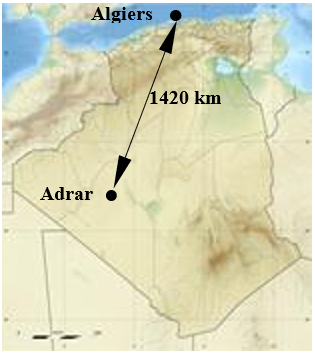(a)

## 1b.png(b)

Figure 1. (a) Adrar location, (b) Annual climograph

## 2.jpg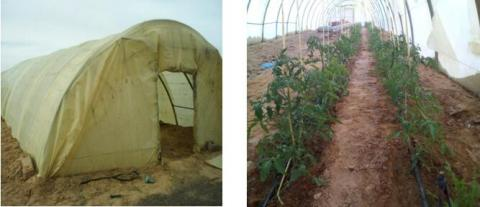Figure 2. Experimental greenhouse

The experimental greenhouse which is the subject of this study is located at the INRAA (Algerian National Institute for Agronomic Research) research station in Adrar. The experimental greenhouse is a tunnel type (Figure 2) occupying a ground surface area of 180 m2 (6 m wide, 30 m long, 3 m high) with a polyethylene cover and houses two rows of tomato plants. The structure is entirely in light galvanized metal tubes. Air renewal in the greenhouse is provided by two doors (1m wide and 2 m high) arranged in opposition on either side of the greenhouse. Irrigation is provided through a drip system controlled by a valve external to the greenhouse.

In our study, the greenhouse cover consists of two rows of tomato plants located in the right part of the greenhouse. The height of each plant is around 1.2 m.

2.2 Temperature measurement

In order to measure the temperature and humidity, two sensors are placed inside the greenhouse. The position of these sensors is shown in Figure 3.

## 3.png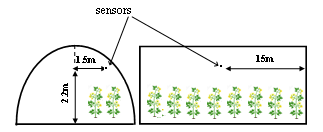Figure 3. Location of temperature/humidity sensors

The campaign of measures was carried out during the month of February of the year 2019, which corresponds to the stage of maturity of the tomato plants. Two types of measurement were carried out outside (Figure 4) inside (Figure 5) and of the greenhouse.

## 4a.png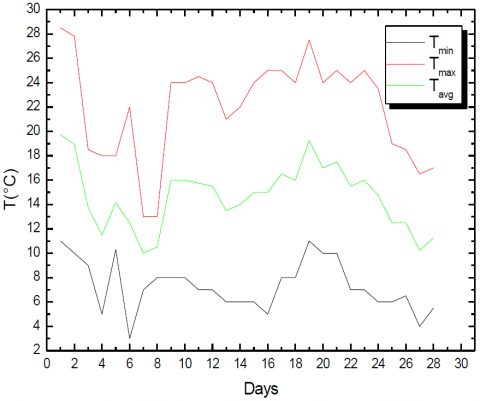Figure 4. February 2019 monthly temperature

## 555.png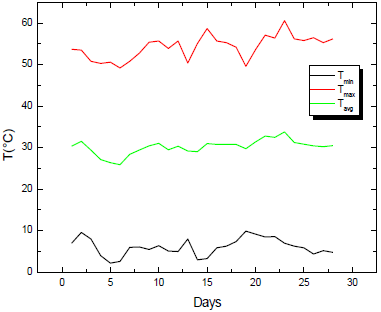Figure 5. Temperature inside the greenhouse

3. Mathematical Formulation

The physical model used in our study is shown in Figure 6.

## 6.pngH=3 m (greenhouse width)

L=6 m (greenhouse length)

e= 0.5 m (plant width)

d= 0.5 m (distandce between rows of plants)

h= 1.2 m (plant height)

Figure 6. Physical model

In order to establish a simple mathematical model that describes the physics of the problem, it is necessary to make a number of simplifying assumptions, namely:

• The fluid is Newtonian and incompressible.
• The environment is continuous.
• The flow regime is laminar and two-dimensional.
• Viscous dissipation is negligible.
• Boussinesq's approximation is validated.

In our study, we will therefore adopt the two-domain approach which consists in writing two systems of equations, one for the fluid medium (Navier-Stokes and energy) and the other for the porous medium (Navier Stokes including the term of Darcy-Brinkman and energy). Taking into account the assumptions made previously, the systems of equations governing our problem is:

3.1 Dimensional equations

• Fluid medium

Continuity equation:

$\frac{\partial u}{\partial x}+\frac{\partial v}{\partial y}=0$             (1)

- Momentum equation along the x axis:

$\rho_{\mathrm{f}}\left(\mathrm{u} \frac{\partial \mathrm{u}}{\partial \mathrm{x}}+\mathrm{v} \frac{\partial \mathrm{u}}{\partial \mathrm{y}}\right)=-\frac{\partial \mathrm{p}}{\partial \mathrm{x}} \mu_{\mathrm{f}}\left(\frac{\partial^{2} \mathrm{u}}{\partial \mathrm{x}^{2}}+\frac{\partial^{2} \mathrm{u}}{\partial \mathrm{y}^{2}}\right)$                    (2)

- Momentum equation along the y axis:

$\rho_{\mathrm{f}}\left(\mathrm{u} \frac{\partial \mathrm{v}}{\partial \mathrm{x}}+\mathrm{v} \frac{\partial \mathrm{v}}{\partial \mathrm{y}}\right)=-\frac{\partial \mathrm{p}}{\partial \mathrm{y}}+\mu_{\mathrm{f}}\left(\frac{\partial^{2} \mathrm{v}}{\partial \mathrm{x}^{2}}+\frac{\partial^{2} \mathrm{v}}{\partial \mathrm{y}^{2}}\right)+\mathrm{g} \beta_{\mathrm{f}}\left(\mathrm{T}-\mathrm{T}_{0}\right)$                       (3)

- Energy equation:

$u \frac{\partial \mathrm{T}}{\partial \mathrm{x}}+v \frac{\partial \mathrm{T}}{\partial \mathrm{y}}=\frac{\lambda_{\mathrm{f}}}{(\rho \mathrm{Cp})_{\mathrm{f}}}\left(\frac{\partial^{2} \mathrm{~T}}{\partial \mathrm{x}^{2}}+\frac{\partial^{2} \mathrm{~T}}{\partial \mathrm{y}^{2}}\right)$                 (4)

• Porous medium

We propose to assimilate plants to a porous medium; moreover, the software for solving the fluid dynamics equations that we use takes into account in a standard way the porous medium type approach with the discretization of the Darcy-Brinkman equations.

- Continuity equation:

$\frac{\partial \mathrm{u}}{\partial \mathrm{x}}+\frac{\partial \mathrm{v}}{\partial \mathrm{y}}=0$

- Momentum equation along the x axis:

$\frac{\rho_{\mathrm{f}}}{\varepsilon^{2}}\left(\mathrm{u} \frac{\partial \mathrm{u}}{\partial \mathrm{x}}+\mathrm{v} \frac{\partial \mathrm{u}}{\partial \mathrm{y}}\right)=-\frac{\partial \mathrm{p}}{\partial \mathrm{x}}+\mu_{\mathrm{eff}} \nabla^{2} \mathrm{u}-\frac{\mu}{\mathrm{K}} \mathrm{u}$                 (5)

- Momentum equation along the y axis:

$\frac{\rho_{\mathrm{f}}}{\varepsilon^{2}}\left(\mathrm{u} \frac{\partial \mathrm{v}}{\partial \mathrm{x}}+\mathrm{v} \frac{\partial \mathrm{v}}{\partial \mathrm{y}}\right)=-\frac{\partial \mathrm{p}}{\partial \mathrm{y}}+\mu_{\mathrm{eff}} \nabla^{2} \mathrm{v}-\frac{\mu}{\mathrm{K}} \mathrm{v}-\rho \mathrm{g}$              (6)

- Energy equation:

$(\rho C p)_{f}\left(u \frac{\partial T}{\partial x}+v \frac{\partial T}{\partial y}\right)=\lambda_{e f f}\left(\frac{\partial^{2} T}{\partial x^{2}}+\frac{\partial^{2} T}{\partial y^{2}}\right)$                 (7)

3.2 Dimensionless equations

The dimensionless form is used in order to find general solutions to physical problems independent of measurement systems. It also allows the simplification of the solution of the systems of equations and the reduction of the physical parameters, so our system of equations is made dimensionless by introducing the following dimensionless variables:

$(\mathrm{X}, \mathrm{Y})=\frac{(\mathrm{x}, \mathrm{y})}{\mathrm{L}} ;(\mathrm{U}, \mathrm{V})=\frac{\mathrm{L}}{\alpha_{\mathrm{f}}}(\mathrm{u}, \mathrm{v}) ; \theta=\frac{\mathrm{T}-\mathrm{T}_{0}}{\Delta \mathrm{T}} ; \mathrm{P}=\frac{\mathrm{pL}^{2}}{\rho_{\mathrm{f}} \alpha_{\mathrm{f}}^{2}}$

• Fluid medium

- Continuity equation:

$\frac{\partial \mathrm{U}}{\partial \mathrm{X}}+\frac{\partial \mathrm{V}}{\partial \mathrm{Y}}=0$

- Momentum equation along the X axis:

$\rho_{\mathrm{f}}\left(\mathrm{U} \frac{\partial \mathrm{U}}{\partial \mathrm{X}}+\mathrm{V} \frac{\partial \mathrm{U}}{\partial \mathrm{Y}}\right)=-\frac{\partial \mathrm{P}}{\partial \mathrm{X}}+\operatorname{Pr}\left(\frac{\partial^{2} \mathrm{U}}{\partial \mathrm{X}^{2}}+\frac{\partial^{2} \mathrm{U}}{\partial \mathrm{Y}^{2}}\right)$                 (8)

- Momentum equation along the Y axis:

$\rho_{\mathrm{f}}\left(\mathrm{U} \frac{\partial \mathrm{V}}{\partial \mathrm{X}}+\mathrm{V} \frac{\partial \mathrm{V}}{\partial \mathrm{Y}}\right)=-\frac{\partial \mathrm{P}}{\partial \mathrm{Y}}+\operatorname{Pr}\left(\frac{\partial^{2} \mathrm{~V}}{\partial \mathrm{X}^{2}}+\frac{\partial^{2} \mathrm{~V}}{\partial \mathrm{Y}^{2}}\right)+\operatorname{RaPr} \theta$               (9)

- Energy equation:

$\mathrm{U} \frac{\partial \theta}{\partial \mathrm{X}}+\mathrm{V} \frac{\partial \theta}{\partial \mathrm{Y}}=\frac{\partial^{2} \theta}{\partial \mathrm{X}^{2}}+\frac{\partial^{2} \theta}{\partial \mathrm{Y}^{2}}$                   (10)

• Porous medium

- Continuity equation:

$\frac{\partial \mathrm{U}}{\partial \mathrm{X}}+\frac{\partial \mathrm{V}}{\partial \mathrm{Y}}=0$

- Momentum equation along the X axis:

$\frac{1}{\varepsilon^{2}}\left(\mathrm{U} \frac{\partial \mathrm{U}}{\partial \mathrm{X}}+\mathrm{V} \frac{\partial \mathrm{U}}{\partial \mathrm{Y}}\right)=-\frac{\partial \mathrm{P}}{\partial \mathrm{X}}+\mathrm{R} \cdot \operatorname{Pr}\left(\frac{\partial^{2} \mathrm{U}}{\partial \mathrm{X}^{2}}+\frac{\partial^{2} \mathrm{U}}{\partial \mathrm{Y}^{2}}\right)-\frac{\operatorname{Pr}}{\mathrm{Da}} \mathrm{U}+\operatorname{RaPr} \theta$              (11)

- Momentum equation along the Y axis:

$\frac{1}{\varepsilon^{2}}\left(\mathrm{U} \frac{\partial \mathrm{V}}{\partial \mathrm{X}}+\mathrm{V} \frac{\partial \mathrm{V}}{\partial \mathrm{Y}}\right)=-\frac{\partial \mathrm{P}}{\partial \mathrm{Y}}+\mathrm{R} \cdot \operatorname{Pr}\left(\frac{\partial^{2} \mathrm{~V}}{\partial \mathrm{X}^{2}}+\frac{\partial^{2} \mathrm{~V}}{\partial \mathrm{Y}^{2}}\right)-\frac{\mathrm{Pr}}{\mathrm{Da}} \mathrm{V}+\mathrm{RaPr} \theta$               (12)

- Energy equation:

$\mathrm{U} \frac{\partial \theta}{\partial \mathrm{X}}+\mathrm{V} \frac{\partial \theta}{\partial \mathrm{Y}}=\lambda_{\mathrm{r}}\left(\frac{\partial^{2} \theta}{\partial \mathrm{X}^{2}}+\frac{\partial^{2} \theta}{\partial \mathrm{Y}^{2}}\right)$                (13)

The dimensionless parameters characterizing our problem are:

$\operatorname{Pr}=\frac{v_{\mathrm{f}}}{\alpha_{\mathrm{f}}} ; \mathrm{Ra}=\frac{\mathrm{g} \beta \mathrm{L}^{3} \Delta \mathrm{T}}{v_{\mathrm{f}} \alpha_{\mathrm{f}}} ; \mathrm{Da}=\frac{\mathrm{K}}{\mathrm{L}^{2}} ; \lambda_{\mathrm{r}}=\frac{\lambda_{\mathrm{m}}}{\lambda_{\mathrm{f}}} ; \mathrm{R}=\frac{\mu_{\mathrm{eff}}}{\mu_{\mathrm{f}}}$

• Boundary conditions

Table 1 includes the hydrodynamic and thermal boundary conditions used in this study and which correspond to the climatic conditions of the Adrar region in winter.

Table 1. Boundary conditions

 Wall Velocity Temperature Superior (blanket) u=v=0 q=0 Lower (ground) u=v=0 q=1 Fluid / Plant interface Continuity Continuity
4. Results and Discussion

In order to solve the system of partial differential Eqns. (1), (8)-(10) in fluid medium and (1), (11)-(13) in porous medium, we used the Galerkin solution method. We tried several meshes and we opted for a mesh comprising 5600 elements. Figure 7 represents the mesh used and which is a linear triangular mesh.

## 777.jpgThe results from the numerical simulation of natural convection in the greenhouse which is the subject of this study are presented in order to determine the flow and heat transfer within the greenhouse.

4.1 Isotherms and streamlines

Figures 8 and 9 represent the streamlines (below) and the isotherms (above) for a Darcy number Da = 0.002, a porosity $\varepsilon$ = 0.531 and for different values of the Rayleigh number. For low values of the Rayleigh number (Ra=103 and Ra=104), the isotherms represent lines parallel to each other thus promoting heat transfer by conduction. There is hardly any movement of the air either far or near the plant. For the other values of the Rayleigh number (Ra=105 and Ra=106), the air flow becomes intense as the latter increases giving rise to heat transfer by convection in the greenhouse.

Whatever the value of Rayleigh number, the structure of the streamlines is characterized by two main counter-rotating cells, the one on the right is located above the plant. By increasing the value of Rayleigh number, this cell widens to the detriment of the one on the left. Air circulation around the two rows of plants increases. This circulation gives freshness to the plant promoting the triggering of biochemical processes.

## 8a.png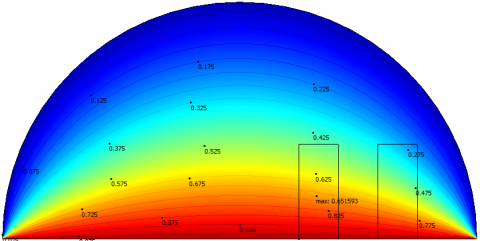## 8a1.png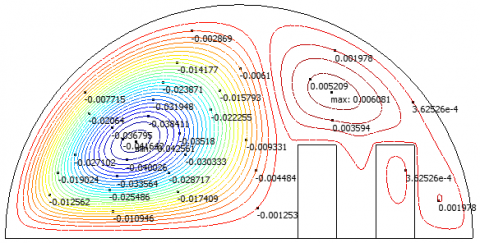(a)

## 8b1.png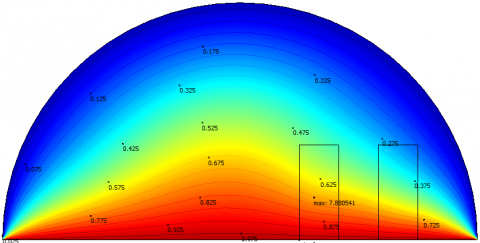## 8b2.png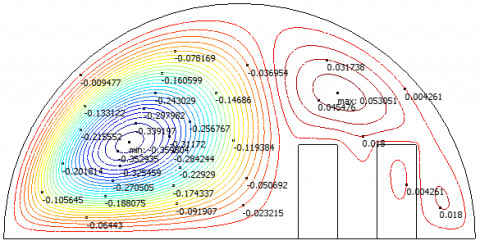(b)

Figure 8. Isotherms and streamlines for Da=0.002; $\varepsilon$=0.531 (a) Ra=103 – (b) Ra=104

## 9a.png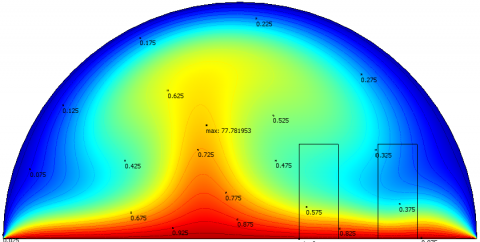## 91b.png(a)

## 9b1.png## 9b2.png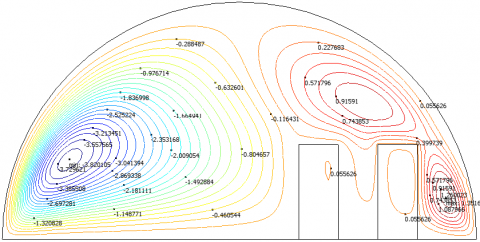(b)

Figure 9. Isotherms and streamlines for Da=0.002; $\varepsilon$=0.531 (a) Ra=105 – (b) Ra=106

4.2 Temperature evolution

The Figure 10 represents the variation of temperature along the horizontal of the greenhouse passing through the mid-height of the plant (Y=0.1).

For low values of the Rayleigh number (Ra=103 and Ra=104), the shape of the curve is the same, noting a maximum at the level of the axis of symmetry (X=0). These low Rayleigh number values have little influence on the temperature distribution within the greenhouse. For the other values of the Rayleigh number (Ra=105 and Ra=106), both curves have an extremum (maximum). The maximum temperature is little influenced by the value of the Rayleigh number. The axis of symmetry of these curves coincides with the axis of symmetry of the greenhouse. The curve loses its symmetry as Rayleigh number decreases.

The Figure 11 represents the evolution of the temperature along the median which presents the axis of symmetry of the greenhouse (X = 0). Whatever the value of Ra, the temperature decreases going from the bottom (ground) to the top (roof). For low values of Rayleigh number (Ra=103 and Ra=104), the decrease in temperature is almost linear. It loses its linearity for the other values of Rayleigh number (Ra=105 and Ra=106).

## 1000.jpg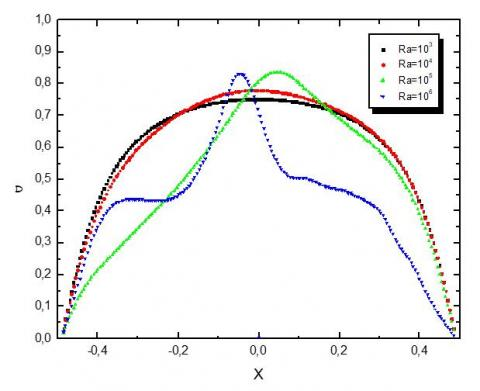Figure 10. $\theta$ vs X for Y=0.1; Da=0.002; $\varepsilon$=0.531

This decrease in temperature from bottom to top gives a warmer environment below the leaves of the plant than the top giving a favorable climate for the growth of the tomato plant.

The Figure 12 represents the temperature evolution along the vertical passing through the middle between the two rows of plants where the temperature sensor has been placed.

## 1111.jpg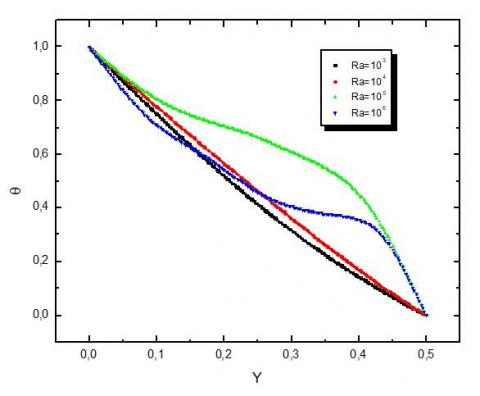Figure 11. $\theta$ vs Y for X=0; Da=0.002; $\varepsilon$=0.531

## 1222.jpg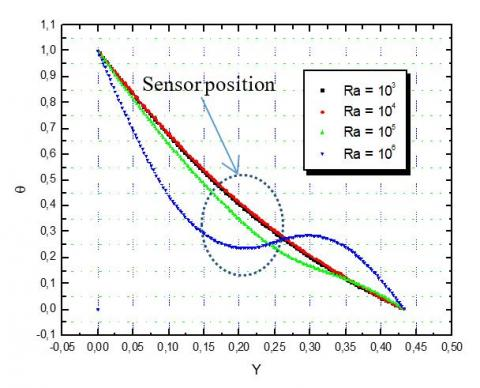Figure 12. $\theta$ vs Y for X=0.25; Da=0.002; $\varepsilon$=0.531

4.3 Velocity Field and stream function

Figure 13 shows the evolution of the vertical component of the velocity along the vertical representing the line of symmetry of the greenhouse. For low Rayleigh number values, the velocity is almost zero, thus promoting conduction. For other Rayleigh number values, the velocity increases with increasing of Rayleigh number. The velocity is maximum at half height of the greenhouse.

## 1333.jpg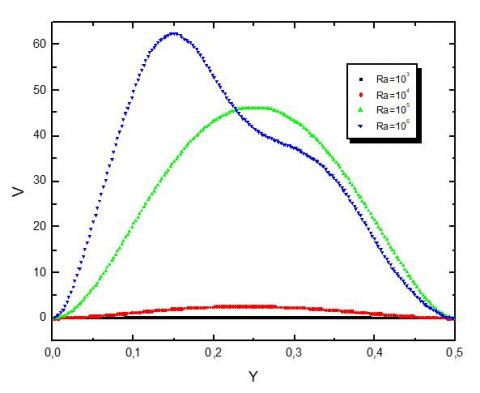Figure 13. V vs Y for X=0; Da=0.002; $\varepsilon$=0.531

Figure 14 shows the variation of the maximum current function as a function of the number of Ra. She believes in a parabolic way. This growth is represented by the correlation given by Eq. (14) with a square of the correlation coefficient R2 is of the order of unity.

## 1444.jpgFigure 14. abs($\psi$)max vs Ra For Da=0.002; $\varepsilon$=0.531

${{a b s}}(\psi)_{\max }=a \cdot R a^{2}+b \cdot R a+c$            (14)

a, b and c represent constants, with:

a=3.44; b= -23.64; c=40.03; R2=0.99998

5. Conclusion

The greenhouse which is the subject of this study is located in the region of Adrar in southwest Algeria. This greenhouse is intended for experimentation in the agricultural field.

In this work, we conducted two types of research:

1- On the one hand, the experimental part of this work focuses on determining the climatic parameters favorable to the growth of the plant and more particularly to that of the tomato plant. For this reason, we have placed sensors to measure the temperature and humidity inside the greenhouse.

2- On the other hand, the theoretical part relates to the numerical resolution by the finite element method of the system of partial differential equations governing the phenomenon of heat transfer and the flow of air within the said greenhouse. The results found show that, for low values of the Rayleigh number, the heat transfer is almost conductive, which takes place at the level of the plant, thus heating the constituents of the latter. While for large Rayleigh numbers, heat transfer by convection becomes dominant which increases the intensity of the air flow and causes it to circulate even around the plant.

In conclusion, to have a favorable environment for the growth of tomatoes, we must keep the openings closed especially during the night without needing a heating system, especially in this region characterized by a hyper arid climate. The value of the temperature calculated by numerical simulation at the position where the sensor has been placed will be compared with that measured by the sensor and which is the subject of another work which will be the continuity of this one followed by a study during the summer period.

Acknowledgement

We gratefully acknowledge the General Directorate of Scientific Research and Technological Development (DGRSDT) for its financial support and unwavering encouragement.

Nomenclature
 Cp Specific heat, J. kg-1. K-1 Da Darcy number g gravitational acceleration, m.s-2 d distance between rows of plants, m e plant width, m h plant height m H greenhouse height, m K Permeability, m-2 L Greenhouse width, m p Dimensional pression, N.m-2 P Dimensionless pression Pr Prandlt number R Dynamic viscosity ratio, R=meff/mf Ra Rayleigh number T Dimensional temperature, ℃ u,v Dimensional Components velocity, m.s-1 U,V Dimensionless Components velocity x,y Dimensional coordinates, m X,Y Dimensionless coordinates Greek symbols a thermal diffusivity, m2. s-1 b thermal expansion coefficient, K-1 µ Dynamic viscosity, kg. m-1.s-1 e Porosity q Dimensionless temperature r Density, kg.m-3 l Thermal conductivity, W.m-1. K-1 Indices f fluid eff effective m matrix
References

 Haxaire, R. (1999). Caracterisation and modeling of air flow in a greenhouse. Ph D thesis, Faculte des Sciences, Universite de Nice Sophie Antipolis, France, pp. 1-148.

 Boulard, T., Haxaire, R., Lamrani, M.A., Roy, J.C., Jaffrin, A. (1999). Characterization and modeling of the air fluxes induced by natural ventilation in a greenhouse. Journal of Agricultural Engineering Research, 74(2): 135-144.

 Draoui, B., Benyamine, M., Touhami, Y., Tahri, B. (1999). Numerical simulation of natural convection in transient laminar regime in a bottom heated tunnel greenhouse (Flux). Journal of Renewable Energies, 141-145. https://www.cder.dz/vlib/revue/nspeciauxpdf/jnv_28.pdf.

 Wang, S., Boulard, T., Haxaire, R. (1999). Air speed profiles in a naturally ventilated greenhouse with a tomato crop. Agricultural and Forest Meteorology, 96: 181-188.

 Ould Khaoua, S.A., Bournet, P.E., Migeon, C., Chassériaux, G., Boulard, T. (2006). Analysis of greenhouse ventilation efficiency with CFD. Bio-Sys Engineering, 95(1): 83-98. https://doi.org/10.1016/j.biosystemseng.2006.05.004

 Bougoul, S., Zeroual, S., Boulard, T., Azil, F. (2007). Numerical simulation of air movement and temperature variation in heated greenhouses. Journal of Renewable Energies, 209-212, CER’07, Oujda, Morroco. https://www.cder.dz/download/cer07_47.pdf.

 Baxevanou, C., Fidaros, D., Bartzanas, T., Kittas, C. (2010). Numerical simulation of solar radiation, air flow and temperature distribution in a naturally ventilated tunnel greenhouse. Agric. Eng. Int.: CIGR Journal, 12(3): 48-67.

 Bartzanas, T., Kittas, C., Tadj, N., Draoui, B. (2007). Influence of vents type arrangement on greenhouse thermal driven ventilation. 2nd PALENC Conference and 28th AIVC Conference on Building Low Energy Cooling and 111 Advanced Ventilation Technologies in the 21st Century, Crete Island, Greece. https://www.aivc.org/sites/default/files/members_area/medias/pdf/Conf/2007/PalencAIVC2007_ 023.pdf.

 Kruger, S., Pretorius, L. (2016). Heat transfer in two and three-dimensional single span greenhouses. 10th South African Conference on Computational and Applied Mechanics.

 Elashmawy, M., Al-Rashed, A.A.A.A., Kolsi, L., Badawy, I.A.Q., Ali, N.B., Ali, S.S. (2017). Heat transfer and fluid flow in naturally ventilated greenhouses. Engineering, Technology & Applied Science Research, 7(4): 1850-1854. https://doi.org/10.48084/etasr.1269

 Mesmoudi, K., Meguellati, K., Bournet, P.E. (2017). Thermal analysis of greenhouses installed under semi-arid climate. International Journal of Heat and Technology. 35(3): 474-486. https://doi.org/10.18280/ijht.350304

  Shivayogi Swamy, K.M., Praveen Kumar, M., Santosh Kumar, K. (2017). Numerical analysis of airflow and temperature distribution in a naturally ventilated greenhouse. Journal of Research in Science, Technology, Engineering and Management, 79-86.

 Raza, S.S., El Kadi, K., Janajreh, I. (2018). Greenhouse microclimate flow simulation: influence of inlet flow conditions. Int. J. of Thermal & Environmental Engineering, 17(1): 11-18. https://doi.org/10.5383/ijtee.17.01.002

 Ouarhlent, F., Soudani, A. (2019). Numerical study of thermal convection in a porous medium. Instrumentation Mesure Metrologie, 18(1): 69-74. https://doi.org/10.18280/i2m.180111

 Ghani, S., El-Bialy, E.M.A.A., Bakochristou, F., Rashwan, M.M., Abdelhalim, A.M., Ismail, S.M., Ben, P. (2020). Experimental and numerical investigation of the thermal performance of evaporative cooled greenhouses in hot and arid climates. Science and Technology for the Built Environment, 26(2): 141-160. https://doi.org/10.1080/23744731.2019.1634421

 Ghernaout, B., Attia, M.E., Bouabdallah, S., Driss, Z., Benali, M.L. (2020). Heat and fluid flow in an agricultural greenhouse. International Journal of Heat and Technology, 38(1): 92-98. https://doi.org/10.18280/ijht.380110

 Jehhef, K.A., Khdair, M.A.S., Thajeel, K.J. (2020). Numerical modeling of thermal radiation heat transfers in agricultural greenhouse. The Fourth Postgraduate Engineering Conference. IOP Conf. Series: Materials Science and Engineering, 745: 012072. https://doi.org/10.1088/1757-899X/745/1/0120721